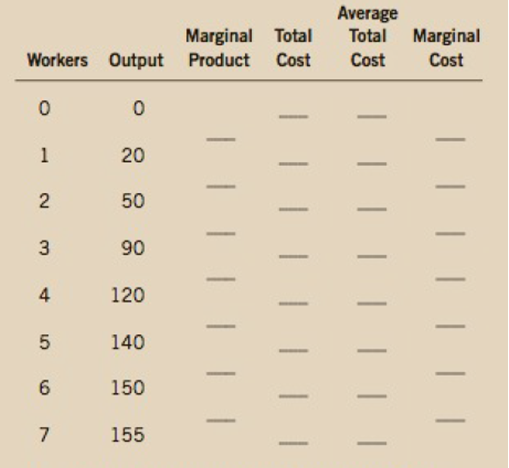Chapter 13, Problem 4PA

Chapter
Section
Textbook Problem

Nimbus, Inc., makes brooms and then sells them door-to-door. Here is the relationship between the number of workers and Nimbus's output during a given day:a. Fill in the column of marginal products. What pattern do you see? How might you explain it?b. A worker costs $100 a day, and the firm has fixed costs of$200. Use this information to fill in the column for total cost.  c Fill in the column for average total cost. (Recall that ΔTC = TC/Q.) What pattern do you see?d. Now fill in the column for marginal cost. (Recall that MC = Δ7C/AQ.) What pattern do you see?e. Compare the column for marginal product with the column for marginal cost. Explain the relationship.f. Compare the column for average total cost with the column for marginal cost. Explain the relationship.

Subpart (a):

To determine
Calculate marginal product.

Explanation

Table -1 shows the data of hours spent for fishing and the quantity of fish caught.

Table -1

 Workers Output Marginal product Total cost Average total cost Marginal cost 0 0 1 20 2 50 3 90 4 120 5 140 6 150 7 155

Marginal product can be calculated by using the following formula:

Marginal product=(OutputPresentOutputPreviousLabor

Subpart (b):

To determine
Calculate Total cost.

Subpart (c):

To determine
Calculate average total cost.

Subpart (d):

To determine
Calculate marginal cost.

Subpart (e):

To determine
Relationship between marginal product and marginal cost.

Subpart (f):

To determine
Relationship between average cost and marginal cost.

Still sussing out bartleby?

Check out a sample textbook solution.

See a sample solution

The Solution to Your Study Problems

Bartleby provides explanations to thousands of textbook problems written by our experts, many with advanced degrees!

Get Started